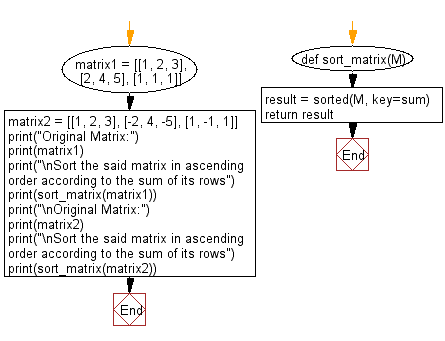﻿ Python: Sort a given matrix in ascending order according to the sum of its rows - w3resource# Python: Sort a given matrix in ascending order according to the sum of its rows

## Python List: Exercise - 101 with Solution

Write a Python program to sort a given matrix in ascending order according to the sum of its rows.

Sample Solution:

Python Code:

``````def sort_matrix(M):
result = sorted(M, key=sum)
return result

matrix1 = [[1, 2, 3], [2, 4, 5], [1, 1, 1]]
matrix2 = [[1, 2, 3], [-2, 4, -5], [1, -1, 1]]

print("Original Matrix:")
print(matrix1)
print("\nSort the said matrix in ascending order according to the sum of its rows")
print(sort_matrix(matrix1))
print("\nOriginal Matrix:")
print(matrix2)
print("\nSort the said matrix in ascending order according to the sum of its rows")
print(sort_matrix(matrix2))
```
```

Sample Output:

```Original Matrix:
[[1, 2, 3], [2, 4, 5], [1, 1, 1]]

Sort the said matrix in ascending order according to the sum of its rows
[[1, 1, 1], [1, 2, 3], [2, 4, 5]]

Original Matrix:
[[1, 2, 3], [-2, 4, -5], [1, -1, 1]]

Sort the said matrix in ascending order according to the sum of its rows
[[-2, 4, -5], [1, -1, 1], [1, 2, 3]]
```

Pictorial Presentation:Flowchart:## Visualize Python code execution:

The following tool visualize what the computer is doing step-by-step as it executes the said program:

Python Code Editor:

Have another way to solve this solution? Contribute your code (and comments) through Disqus.

What is the difficulty level of this exercise?

Test your Python skills with w3resource's quiz

﻿

## Python: Tips of the Day

```print(2_000_000)
```2000000